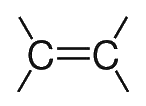Search:

# The geometry of organic molecules

- In alkanes the $C$ atom has a tetrahedral geometry:Thus, there is only one dichloromethane:- In alkenes the $C$ atom has a rigid planar geometry:Thus, there are three dichloroethenes:- In alkynes the $C$ atom has a linear geometry: